## f(x) = 4x^2 + 7x – 18 Find f(-9)

Question

f(x) = 4x^2 + 7x – 18
Find f(-9)

in progress 0
3 weeks 2021-09-01T13:39:49+00:00 2 Answers 0 views 0

1. ### Given :

• f(x) = 4x² + 7x – 18

• f(- 9) = ?

### Solution :

As, we have f(x) = 4x² + 7x – 18, so to find f(- 9) substitute value of x as (-9).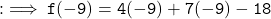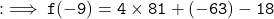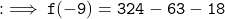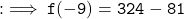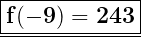Hence, value of f(- 9) = 243.

f(-9) = 243

General Formulas and Concepts:

Pre-Algebra

Order of Operations: BPEMDAS

1. Brackets
2. Parenthesis
3. Exponents
4. Multiplication
5. Division
7. Subtraction
• Left to Right

Algebra I

• Function Notations

Step-by-step explanation:

Step 1: Define

f(x) = 4x² + 7x – 18

f(-9) is x = -9

Step 2: Evaluate

1. Substitute in x:                                                                                                f(-9) = 4(-9)² + 7(-9) – 18
2. Evaluate exponents:                                                                                      f(-9) = 4(81) + 7(-9) – 18
3. Multiply:                                                                                                           f(-9) = 324 – 63 – 18
4. Subtract:                                                                                                          f(-9) = 261 – 18
5. Subtract:                                                                                                          f(-9) = 243